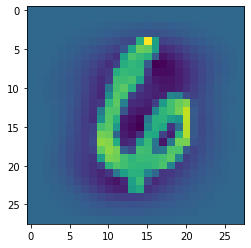#### Logistic Regression

p
R
```#!/usr/bin/python

# Logistic Regression from scratch

# In:

# In:

# importing all the required libraries

"""
Implementing logistic regression for classification problem
Coursera ML course
https://medium.com/@martinpella/logistic-regression-from-scratch-in-python-124c5636b8ac
"""
import numpy as np
from matplotlib import pyplot as plt
from sklearn import datasets

# get_ipython().run_line_magic('matplotlib', 'inline')

# In:

# sigmoid function or logistic function is used as a hypothesis function in
# classification problems

def sigmoid_function(z: float | np.ndarray) -> float | np.ndarray:
"""
Also known as Logistic Function.

1
f(x) =   -------
1 + e⁻ˣ

The sigmoid function approaches a value of 1 as its input 'x' becomes
increasing positive. Opposite for negative values.

Reference: https://en.wikipedia.org/wiki/Sigmoid_function

@param z:  input to the function
@returns: returns value in the range 0 to 1

Examples:
>>> sigmoid_function(4)
0.9820137900379085
>>> sigmoid_function(np.array([-3, 3]))
array([0.04742587, 0.95257413])
>>> sigmoid_function(np.array([-3, 3, 1]))
array([0.04742587, 0.95257413, 0.73105858])
>>> sigmoid_function(np.array([-0.01, -2, -1.9]))
array([0.49750002, 0.11920292, 0.13010847])
>>> sigmoid_function(np.array([-1.3, 5.3, 12]))
array([0.21416502, 0.9950332 , 0.99999386])
>>> sigmoid_function(np.array([0.01, 0.02, 4.1]))
array([0.50249998, 0.50499983, 0.9836975 ])
>>> sigmoid_function(np.array([0.8]))
array([0.68997448])
"""
return 1 / (1 + np.exp(-z))

def cost_function(h: np.ndarray, y: np.ndarray) -> float:
"""
Cost function quantifies the error between predicted and expected values.
The cost function used in Logistic Regression is called Log Loss
or Cross Entropy Function.

J(θ) = (1/m) * Σ [ -y * log(hθ(x)) - (1 - y) * log(1 - hθ(x)) ]

Where:
- J(θ) is the cost that we want to minimize during training
- m is the number of training examples
- Σ represents the summation over all training examples
- y is the actual binary label (0 or 1) for a given example
- hθ(x) is the predicted probability that x belongs to the positive class

@param h: the output of sigmoid function. It is the estimated probability
that the input example 'x' belongs to the positive class

@param y: the actual binary label associated with input example 'x'

Examples:
>>> estimations = sigmoid_function(np.array([0.3, -4.3, 8.1]))
>>> cost_function(h=estimations,y=np.array([1, 0, 1]))
0.18937868932131605
>>> estimations = sigmoid_function(np.array([4, 3, 1]))
>>> cost_function(h=estimations,y=np.array([1, 0, 0]))
1.459999655669926
>>> estimations = sigmoid_function(np.array([4, -3, -1]))
>>> cost_function(h=estimations,y=np.array([1,0,0]))
0.1266663223365915
>>> estimations = sigmoid_function(0)
>>> cost_function(h=estimations,y=np.array())
0.6931471805599453

References:
- https://en.wikipedia.org/wiki/Logistic_regression
"""
return (-y * np.log(h) - (1 - y) * np.log(1 - h)).mean()

def log_likelihood(x, y, weights):
scores = np.dot(x, weights)
return np.sum(y * scores - np.log(1 + np.exp(scores)))

# here alpha is the learning rate, X is the feature matrix,y is the target matrix
def logistic_reg(alpha, x, y, max_iterations=70000):
theta = np.zeros(x.shape)

for iterations in range(max_iterations):
z = np.dot(x, theta)
h = sigmoid_function(z)
gradient = np.dot(x.T, h - y) / y.size
theta = theta - alpha * gradient  # updating the weights
z = np.dot(x, theta)
h = sigmoid_function(z)
j = cost_function(h, y)
if iterations % 100 == 0:
print(f"loss: {j} \t")  # printing the loss after every 100 iterations
return theta

# In:

if __name__ == "__main__":
import doctest

doctest.testmod()

x = iris.data[:, :2]
y = (iris.target != 0) * 1

alpha = 0.1
theta = logistic_reg(alpha, x, y, max_iterations=70000)
print("theta: ", theta)  # printing the theta i.e our weights vector

def predict_prob(x):
return sigmoid_function(
np.dot(x, theta)
)  # predicting the value of probability from the logistic regression algorithm

plt.figure(figsize=(10, 6))
plt.scatter(x[y == 0][:, 0], x[y == 0][:, 1], color="b", label="0")
plt.scatter(x[y == 1][:, 0], x[y == 1][:, 1], color="r", label="1")
(x1_min, x1_max) = (x[:, 0].min(), x[:, 0].max())
(x2_min, x2_max) = (x[:, 1].min(), x[:, 1].max())
(xx1, xx2) = np.meshgrid(np.linspace(x1_min, x1_max), np.linspace(x2_min, x2_max))
grid = np.c_[xx1.ravel(), xx2.ravel()]
probs = predict_prob(grid).reshape(xx1.shape)
plt.contour(xx1, xx2, probs, [0.5], linewidths=1, colors="black")

plt.legend()
plt.show()
```
``````import numpy as np
import pandas as pd
import sklearn``````

### Importing the MNIST Dataset to perform Multi-Class Classification.

MNIST is a large database of handwritten digits that is commonly used for training various image processing systems.

``````from sklearn.datasets import fetch_openml
X, y = fetch_openml('mnist_784', return_X_y=True)``````

### Shape of the dataset

``X.shape``
`(70000, 784)`

### Visualizing the first 5 images in the dataset

We are reshaping the each array as a 28X28 Image so that we can plot it

``````import matplotlib.pyplot as plt
for i in range(5):
plt.imshow(X[i].reshape((28,28)))
plt.show()``````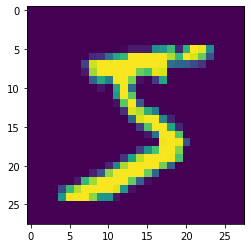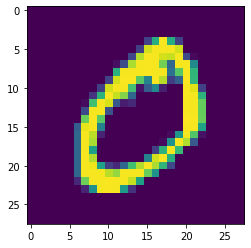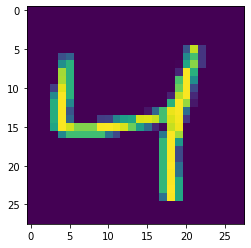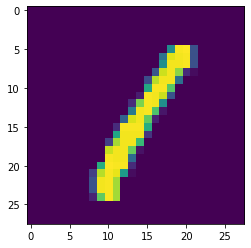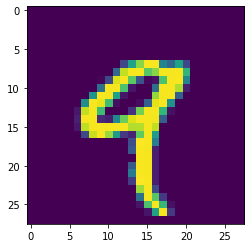## Importing the Logistic Regression Model from Scikit-Learn and Data Processing

1. We are decreasing the size of the dataset for training.
2. Then, we scale the data.
3. Finally, we split the dataset into training and test sets
``````from sklearn.linear_model import LogisticRegression
from sklearn.model_selection import train_test_split
from sklearn import preprocessing

# STEP 1
X, y = X[:10_000], y[:10_000]

# STEP 2
X_scaled = preprocessing.scale(X)

# STEP 3
X_train, X_test, y_train, y_test = train_test_split(X_scaled, y, random_state = 42)``````

## Then, we finally train the Logistic Regression Model.

``````model = LogisticRegression(random_state=0, max_iter=2000)
model.fit(X_train, y_train)
print("Model Training Completed!")``````
```Model Training Completed!
```

### We now measuring the accuracy score of the model that we have trained.

``````from sklearn.metrics import accuracy_score
preds = model.predict(X_test)
score = accuracy_score(y_test, preds)
print(f"Model's accuracy score is : {round(score*100, 2)} %")``````
```Model&#x27;s accuracy score is : 89.76 %
```

### Demonstrating the model's predictions on one of the image from our test-set.

``````pred = model.predict(X_test.reshape(-1, 784))
print(f"Model's Prediction : {pred}")
print("*"*30)

plt.imshow(X_test.reshape(28, 28))
plt.show()``````
```Model&#x27;s Prediction : [&#x27;6&#x27;]
******************************
```